# Exercise E11.2 Constructions NCERT Solutions Class 9

## Chapter 11 Ex.11.2 Question 1

Construct a triangle $$ABC$$ in which $$BC =$$ $$7\;\rm{cm}$$ , $$\angle B$$ = $$75°$$ and \begin{align}\mathrm{AB}+\mathrm{AC}=13 \mathrm{cm}\end{align}

### Solution

Steps of Construction: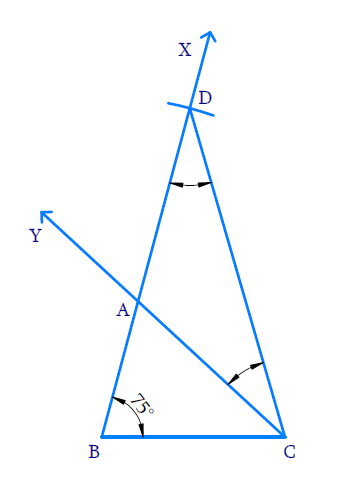(i) Draw base  $$BC =7\;\rm{cm}$$ and at point $$B$$ make an angle of $$75^\circ$$ using protractor.

(ii) With $$B$$ as center and radius equal to $$13\;\rm{cm}$$, draw an arc to intersect ray $$BX$$ at $$D$$.

(iii) Join $$DC$$

(iv) Measure $$\angle \mathrm{D}$$  and make  $$\angle \mathrm{ACD}=\angle \mathrm{D}$$

(v) Let $$CY$$ intersect $$BD$$ at $$A$$

(vi) $$ABC$$ is the required triangle

## Chapter 11 Ex.11.2 Question 2

Construct a triangle $$ABC$$ in which $${BC}=8\;\mathrm{cm}, \angle B=\;45^{\circ}$$ and $$AB-AC=3.5\;\rm{cm}.$$

### Solution

Steps of Construction: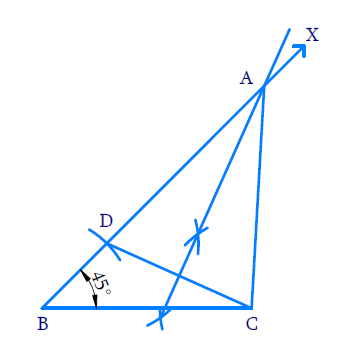(i) Draw base $$BC = 8\;\rm{cm}$$ and at point $$B$$ make an angle of using $$45^{\circ}$$ a protractor.

(ii) With $$B$$ as centre and radius $$BD = 3.5\;\rm{cm}$$, draw an arc to intersect ray $$BX$$ at $$D$$.

(iii) Join $$DC$$

(iv) With $$D$$ and $$C$$ as the centre and radius greater than half of $$DC$$. Draw arcs above and below the line to intersect ray $$BX$$ at $$A$$.

(v) Join $$AC$$.$$ABC$$ is the required triangle.

## Chapter 11 Ex.11.2 Question 3

Construct a triangle $$PQR$$ in which $$QR=6\;\mathrm{cm}, Q=\angle 60^{\circ}$$ and $$PR-PQ= 2\;\rm{cm}.$$

### Solution

Steps of Construction: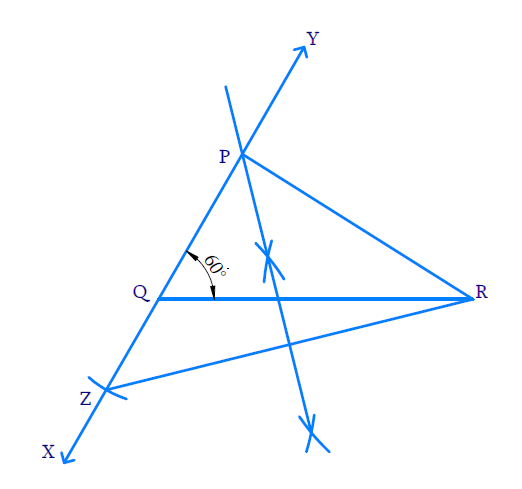(i) Draw line  $$QR =6\;\rm{cm}$$ . Make an angle of $$60^{\circ}$$ at a point $$Q$$ using a protractor and extend it below line $$QR$$.

(ii) With $$Q$$ as center and radius as $$2\;\rm{cm}$$, draw an arc to intersect the ray $$QX$$ at $$Z$$

(iii) Join $$RZ$$

(iv) With $$Z$$ and $$R$$ as centres and radius greater than half of $$ZR$$, draw arcs on either side to intersect each other.

(v) Join the intersecting points and extend it to meet the ray $$QY$$ at $$P$$.

(vi) Join $$P$$ and $$R$$ .$$PQR$$ is the required triangle.

## Chapter 11 Ex.11.2 Question 4

Construct a triangle $$XYZ$$ in which $$\angle Y=30^{\circ}, \angle Z=90^{\circ}$$ and $$XY + YZ + ZX = 11 \;\rm{cm.}$$

### Solution

Steps of Construction: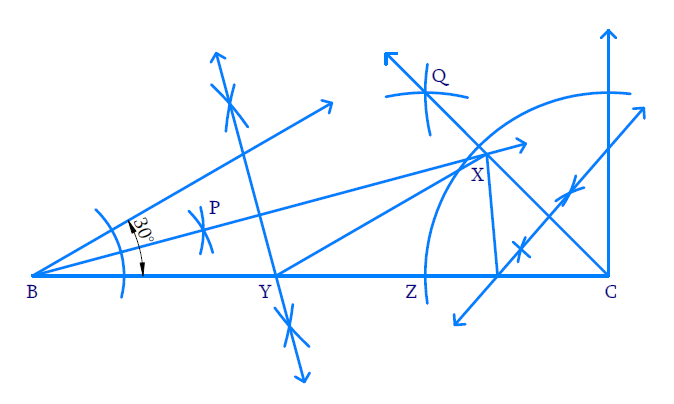(i) Draw line  $$BC=11\;\rm{cm}$$.

(ii) Make angle of $$30^\circ$$ at $$B$$ and $$90^\circ$$ at $$C$$ using a protractor.

(iii) Bisect angle $$B$$.

With $$B$$ as centre and any radius draw a wide arc to intersect both the arms of angle $$B$$.

(iv) With intersecting points as the centre and same radius draw two arcs to intersect each other at $$P$$ Draw line joining $$B$$ and $$P$$ and extend it beyond $$P$$

(v) Bisect angle $$C$$.

With $$C$$ as the centre and radius draw two arcs to intersect each other at $$Q$$. Join $$Q$$ and $$C$$such that it intersects ray $$BP$$ at $$X$$.

(vi) Perpendicular bisector of $$BX$$.

With $$B$$ and $$X$$ as centres and radius greater than half of $$BX$$ draw arcs on either side of line $$BX$$ to intersect each other. Join the intersecting lines such that the perpendicular bisector intersects $$BC$$ at $$Y$$.

(vii) Perpendicular bisector of $$CX$$

With $$C$$ and $$X$$ as centres and radius greater than half of $$CX$$ draw arcs on Join the intersecting lines such that the perpendicular bisector intersects $$BC$$ at $$Z$$.

(viii) Join $$XY$$ and $$XZ$$. $$XYZ$$ is the required triangle.

## Chapter 11 Ex.11.2 Question 5

Construct a right triangle whose base is $$12\;\rm{cm}$$  and sum of its hypotenuse and other side is $$18\;\rm{cm}$$

### Solution

Steps of Construction: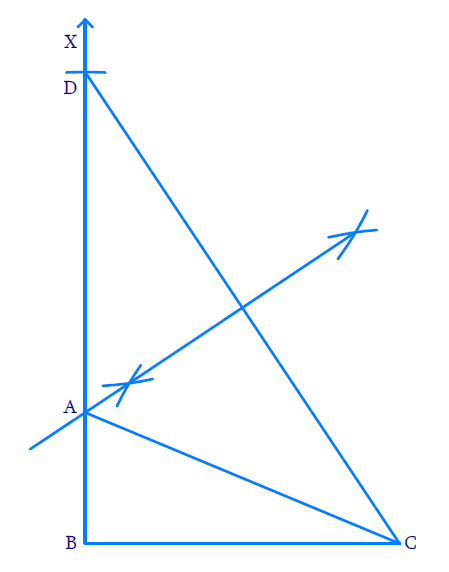(i) Draw the base $$BC=12\;\rm{cm}$$.

(ii) At the point $$B$$, make an angle $$CBX=90^\circ$$ using a protractor.

(iii) Cut a line segment $$BD=18\;\rm{cm}$$ from the ray $$BX$$.

(iv) Join $$DC$$

(v) With $$D$$ and $$C$$ as the centres and radius greater than half of $$DC$$ draw arcs on either side of the line to intersect each other. Join the intersecting points and extend the perpendicular bisector to meet $$BD$$ at $$A$$.

(vi) Join $$A$$ and $$C$$$$ABC$$ is the required right-angled triangle

Instant doubt clearing with Cuemath Advanced Math Program